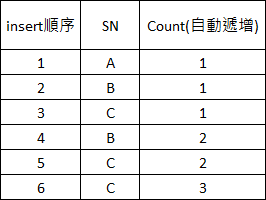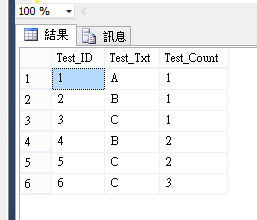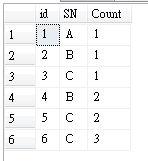0

MS SQL Auto Increment的欄位可否依據某欄位做遞增值?3 個回答

1

declare @Test table(
Test_ID int
,Test_Txt nvarchar(5)
,Test_Count int
)

insert into @Test
select Set_ID
,Set_Txt
,isNull((select count(*) from @Test where Set_ID >= Test_ID and Set_Txt = Test_Txt),0)+1 as Set_Count
from (
select isNull((select max(Test_ID) from @Test),0) + 1 as Set_ID
,'A' as Set_Txt
) as k

insert into @Test
select Set_ID
,Set_Txt
,isNull((select count(*) from @Test where Set_ID >= Test_ID and Set_Txt = Test_Txt),0)+1 as Set_Count
from (
select isNull((select max(Test_ID) from @Test),0) + 1 as Set_ID
,'B' as Set_Txt
) as k

insert into @Test
select Set_ID
,Set_Txt
,isNull((select count(*) from @Test where Set_ID >= Test_ID and Set_Txt = Test_Txt),0)+1 as Set_Count
from (
select isNull((select max(Test_ID) from @Test),0) + 1 as Set_ID
,'C' as Set_Txt
) as k

insert into @Test
select Set_ID
,Set_Txt
,isNull((select count(*) from @Test where Set_ID >= Test_ID and Set_Txt = Test_Txt),0)+1 as Set_Count
from (
select isNull((select max(Test_ID) from @Test),0) + 1 as Set_ID
,'B' as Set_Txt
) as k

insert into @Test
select Set_ID
,Set_Txt
,isNull((select count(*) from @Test where Set_ID >= Test_ID and Set_Txt = Test_Txt),0)+1 as Set_Count
from (
select isNull((select max(Test_ID) from @Test),0) + 1 as Set_ID
,'C' as Set_Txt
) as k

insert into @Test
select Set_ID
,Set_Txt
,isNull((select count(*) from @Test where Set_ID >= Test_ID and Set_Txt = Test_Txt),0)+1 as Set_Count
from (
select isNull((select max(Test_ID) from @Test),0) + 1 as Set_ID
,'C' as Set_Txt
) as k

select * from @Testrchin iT邦新手 5 級 ‧ 2018-11-16 08:48:22 檢舉

1

rchin iT邦新手 5 級 ‧ 2018-11-16 08:50:09 檢舉

1

1.先建一個函數GetSNCount(假設Table Name是dbo.test)

CREATE FUNCTION [dbo].[GetSNCount](@SN varchar(10))

RETURNS  int
AS
BEGIN
Declare @SNCount int = 0
SELECT @SNCount=count(SN) from dbo.test where SN=@SN group by SN

set @SNCount = @SNCount + 1
-- Return the result of the function
RETURN @SNCount

END

2.Insert測試資料

insert test(sn,[count]) values('A',dbo.getSNCount('A'))
insert test(sn,[count]) values('B',dbo.getSNCount('B'))
insert test(sn,[count]) values('C',dbo.getSNCount('C'))
insert test(sn,[count]) values('B',dbo.getSNCount('B'))
insert test(sn,[count]) values('C',dbo.getSNCount('C'))
insert test(sn,[count]) values('C',dbo.getSNCount('C'))

3.結果如下